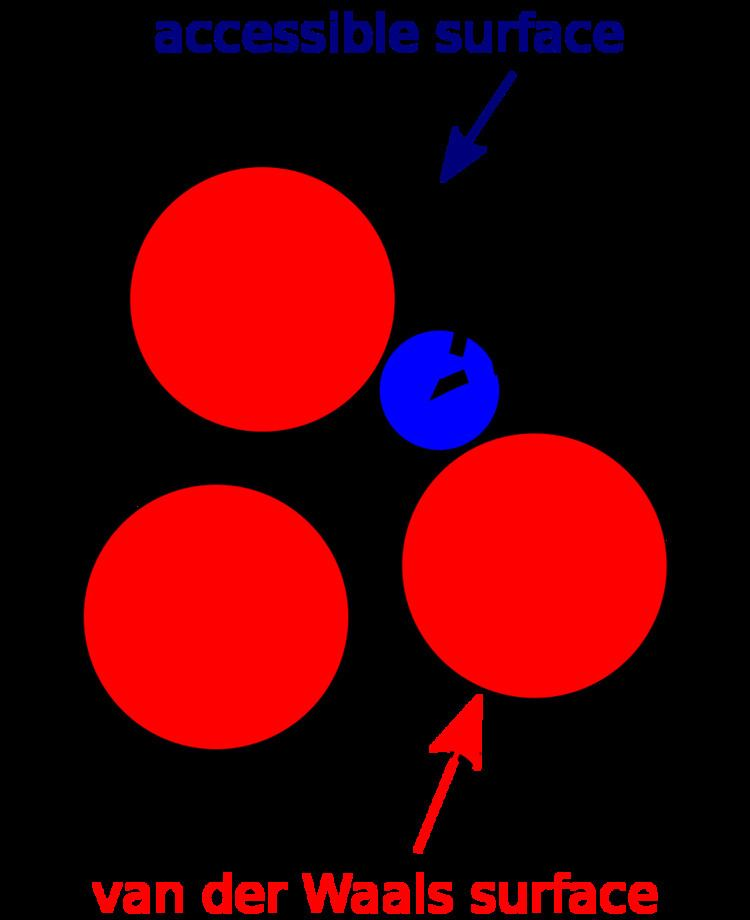# Accessible surface area

Updated on
Covid-19The accessible surface area (ASA) or solvent-accessible surface area (SASA) is the surface area of a biomolecule that is accessible to a solvent. Measurement of ASA is usually described in units of square ångstroms (a standard unit of measurement in molecular biology). ASA was first described by Lee & Richards in 1971 and is sometimes called the Lee-Richards molecular surface. ASA is typically calculated using the 'rolling ball' algorithm developed by Shrake & Rupley in 1973. This algorithm uses a sphere (of solvent) of a particular radius to 'probe' the surface of the molecule.

## Shrake-Rupley algorithm

The Shrake-Rupley algorithm is a numerical method that draws a mesh of points equidistant from each atom of the molecule and uses the number of these points that are solvent accessible to determine the surface area. The points are drawn at a water molecule's estimated radius beyond the van der Waals radius, which is effectively similar to ‘rolling a ball’ along the surface. All points are checked against the surface of neighboring atoms to determine whether they are buried or accessible. The number of points accessible is multiplied by the portion of surface area each point represents to calculate the ASA. The choice of the 'probe radius' does have an effect on the observed surface area, as using a smaller probe radius detects more surface details and therefore reports a larger surface. A typical value is 1.4Å, which approximates the radius of a water molecule. Another factor that affects the results is the definition of the VDW radii of the atoms in the molecule under study. For example, the molecule may often lack hydrogen atoms which are implicit in the structure. The hydrogen atoms may be implicitly included in the atomic radii of the 'heavy' atoms, with a measure called the 'group radii'. In addition, the number of points created on the van der Waals surface of each atom determines another aspect of discretization, where more points provide an increased level of detail.

## LCPO method

The LCPO method uses a linear approximation of the two-body problem for a quicker analytical calculation of ASA. The approximations used in LCPO result in an error in the range of 1-3 Å².

## Power Diagram method

Recently a method was presented that calculates ASA fast and analytically using a power diagram.

## Applications

Accessible surface area is often used when calculating the transfer free energy required to move a biomolecule from aqueous solvent to a non-polar solvent such as a lipid environment. The LCPO method is also used when calculating implicit solvent effects in the molecular dynamics software package AMBER.

It is recently suggested that (predicted) accessible surface area can be used to improve prediction of protein secondary structure.

## Relation to solvent-excluded surface

The ASA is closely related to the concept of the solvent-excluded surface (also known as the molecular surface or Connolly surface), which is imagined as a cavity in bulk solvent (effectively the inverse of the solvent-accessible surface). It is also calculated in practice via a rolling-ball algorithm developed by Frederic Richards and implemented three-dimensionally by Michael Connolly in 1983 and Tim Richmond in 1984. Connolly spent several more years perfecting the method.

Accessible surface area Wikipedia

Topics